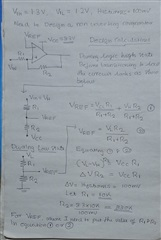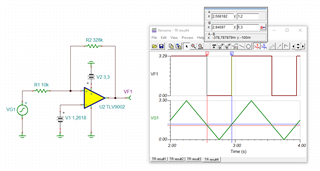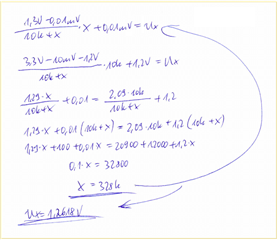If you have a related question, please click the "Ask a related question" button in the top right corner. The newly created question will be automatically linked to this question.

# TLV7011: Comparator with Hysteresis_Design Calculations

Part Number: TLV7011

Dear Team,

I need to design a comparator with Vih=1.3V and Vil=1.2V. Therefore hysteresis is 100mV. The circuit will be working on a 3.3V supply. During high state, the o/p of the comparator will be 3.3V and at the low state, it will be 0V. I will be using a comparator with a push-pull output configuration. Please see my design calculations below.I would like to know two things

1)May I know my design calculation is correct or not.

2)For finding Vref where I need to plug the value of R1 and R2.In equation 1 or 2Regards

HARI

• The R2/R1 factor of 33 is correct.

If the equations were correct, they would result in the same VREF value.

Due to how the switching with hysteresis works, you have to exchange VIH and VIL. Then for both equations, VREF ≈ 1.26 V.

• Thank you.

When I switched  Vih and Vil I am getting the correct Vref.

But I did not understand why I need to switch. I am confused there.

If you don't mind could you please explain dufference betwenn analysis of inverting and non inverting comparator .Taking the same circuit as example

Redards

HARI

• In your calculations, you have considered "during high state" and "during low state". But you need to consider the point in time where the circuit switches between the high and low states, i.e., when the output of the comparator is in the old state, but the input voltage is moving to the new state.

An inverting comparator would use a different circuit (the feedback goes to the positive input, so the feedback current affects the VREF voltage divider). See Inverting Comparator With Hysteresis Circuit.

• Hi Hari,

To add onto Clemens' point, the idea behind hysteresis is to create a window beyond the reference voltage that if an input signal that is near that reference voltage is noisy enough, it might cross over and under that reference voltage unintentionally; causing your output to toggle when you didn't want it to.

Therefore that window is set with VIH and VIL. VIH is the voltage level that your input voltage would need to cross above during an output low state in order to trip the comparator "high". VIL would be the opposite of that, being the voltage level that your input voltage would need to cross below during an output high state in order to trip the comparator "low".

The overall idea is that by implementing the window, the input voltage would need to cross the reference voltage as well as part of the window (up to VIH or VIL) to toggle the comparator output.

Sincerely,

Gerome

•hari_tlv9001.TSCKai

• Hi Cacho,

Thank yoou.

Regards

HARI

• Hi Kai,

Thank you .

Regards

HARI